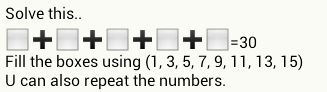# UPSC number Puzzle – ? + ? + ? + ? + ? = 30

1,118.1K Views

? + ? + ? + ? + ? = 30

You have to fill numbers in place of question marks.
The numbers that you can use are 1, 3, 5, 7, 9, 11, 13 and 15.
You can repeat the numbers if you like.

Can you solve it?The question that follows came in the UPSC final exam that was held in December 2013.
Only one examinee(Gaurav Agarwal) was able to solve it.

(15-9)+(13-7)+(7-1)+(9-1)+(13-9)=30

When we calculate it, we get
6 + 6 + 6 + 8 + 4 = 30

30 = 11+9+3+1+3!

3!=3*2*1=6

It seems that something’s missing from the puzzle… Because there are a number of straight-forward answers to  the question in its present form:

1 + 3 + 11 + 15 = 30
1+ 5 + 9 + 15  = 30
3 + 5 + 7 + 15 = 30
1 + 7 + 9 + 13 = 30 etc. being a few, without repeating the numbers.  (With ‘repeat’ option, answers are plenty.)You are asked to express as a sum of 5 numbers!

on 18th May 2016.